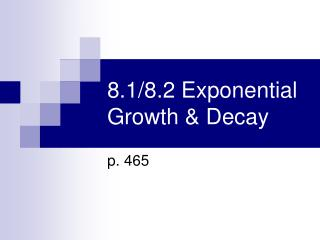DownloadDownload Presentation8.1/8.2 Exponential Growth & Decay

# 8.1/8.2 Exponential Growth & Decay

Télécharger la présentation## 8.1/8.2 Exponential Growth & Decay

- - - - - - - - - - - - - - - - - - - - - - - - - - - E N D - - - - - - - - - - - - - - - - - - - - - - - - - - -
##### Presentation Transcript

1. Exponential Function • f(x) = bx where the base b is a positive number other than one. • Graph f(x) = 2x • Note the end behavior • x→∞ f(x)→∞ • x→-∞ f(x)→0 • y=0 is an asymptote

2. Asymptote • A line that a graph approaches as you move away from the origin The graph gets closer and closer to the line y = 0 ……. But NEVER reaches it 2 raised to any power Will NEVER be zero!! y = 0

3. These are true of y = abx • If a>0 & b>1 ……… • The function is an Exponential Growth Function

4. Example 1 • Graph y = ½ 3x • Plot ordered pairs (0, ½) and (1, 3/2) • Then, from left to right, draw a curve that begins just above the x-axis, passes thru the 2 points, and moves up to the right

5. D= (-∞, +∞) R= (0, +∞) y = 0 Always mark asymptote!!

6. Example 2 y = 0 • Graph y = - (3/2)x • Plot (0, -1) and (1, -3/2) • Connect with a curve • Mark asymptote • D=? • R=?

7. Now…you try one! • Graph y= 2·3x-2 +1 • State the Domain and Range! • D= ? • R= ? y=1

8. Exponential Decay • Has the same form as growth functions f(x) = abx • Where a > 0 • BUT: • 0 < b < 1 (a fraction between 0 & 1)

9. Recognizing growth and decay functions • State whether f(x) is an exponential growth or decay function • f(x) = 5(2/3)x • b=2/3, 0<b<1 it is a decay function. • f(x) = 8(3/2)x • b= 3/2, b>1 it is a growth function. • f(x) = 10(3)-x • rewrite as f(x)=10(1/3)x so it is decay

10. Graph: • y = 3(1/4)x • Plot (0,3) and (1,3/4) • Draw & label asymptote • Connect the dots using the asymptote y=0 Domain = all reals Range = reals>0

11. Graph y=0 • y = -5(2/3)x • Plot (0,-5) and (1,-10/3) • Draw & label asymptote • Connect the dots using the asymptote Domain : all reals Range : y < 0

12. Example graph y= -3(1/2)x+2 + 1 y=1 Domain : Range :

13. Assignment:page 470#30-36 evenPage 478#30-36 even